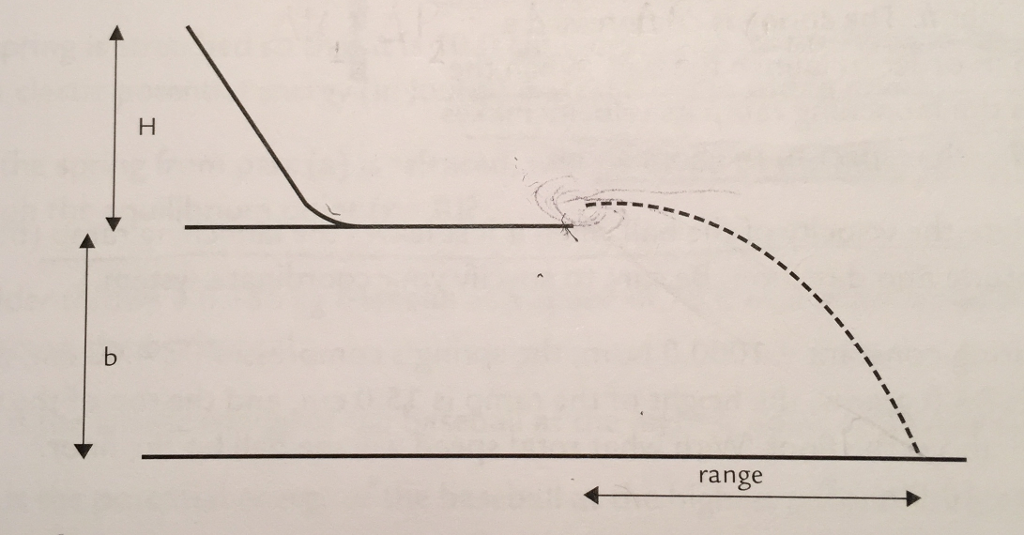# Problem: Please refer to this diagram for the problems listed below.1. Using Conservation of Energy and Kinematics, find an expression (derive) for the range of a ball (launched off of the ramp highlighted in the picture) in terms of H, b, and constants.

###### FREE Expert Solution

Conservation of energy:

$\begin{array}{rcl}\mathbf{K}{\mathbf{E}}_{\mathbf{i}}\mathbf{+}\mathbf{P}{\mathbf{E}}_{\mathbf{i}}& \mathbf{=}& \mathbf{K}{\mathbf{E}}_{\mathbf{f}}\mathbf{+}\mathbf{P}{\mathbf{E}}_{\mathbf{f}}\\ \mathbf{P}{\mathbf{E}}_{\mathbf{i}}& \mathbf{=}& \mathbf{K}{\mathbf{E}}_{\mathbf{f}}\\ \mathbf{m}\mathbf{g}\mathbf{H}& \mathbf{=}& \frac{\mathbf{1}}{\mathbf{2}}\mathbf{m}{\mathbf{v}}^{\mathbf{2}}\\ {\mathbf{v}}_{\mathbf{x}}& \mathbf{=}& \sqrt{\mathbf{2}\mathbf{g}\mathbf{H}}\end{array}$###### Problem Details

Please refer to this diagram for the problems listed below.1. Using Conservation of Energy and Kinematics, find an expression (derive) for the range of a ball (launched off of the ramp highlighted in the picture) in terms of H, b, and constants.